ISEE Middle Level Math : How to add variables

Example Questions

← Previous 1 3 4 5 6

Example Question #4 : Algebra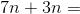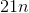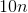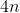Explanation:

Add the numbers and keep the variable: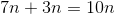Answer:Example Question #5 : Algebra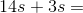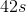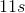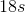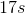Explanation:

Add the numbers and keep the variable: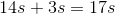Answer:Example Question #6 : Algebra

Simplify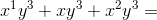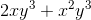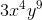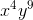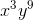Explanation:

In order to add variables the terms must be like. In order for terms to be like, the variables must be exactly alike also being raised to the same power by the exponent.

In this case the like terms are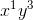and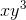. Just because there is a 1 in the exponent for the first term doesnt mean it is different from the second term. With exponents if a variable does not show an exponent, that means it is still to the first power.

We add the coefficients of the like terms. The coefficient is the number in front of the first variable, in this case it is 1 for both terms because of the identity property of multiplication stating any variable, term, or number multiplied by 1 is itself.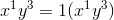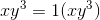Our last term is not like because thevariable is raised to a different power than the other two. In this case we do not combine it to the like terms, we just add it to the end of the term.

Example Question #7 : Algebra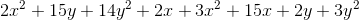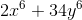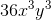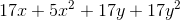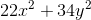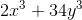Explanation:

Remember, for exponent problems, you group together different exponents and different combinations of variables as though each were a different type of variable.  Therefore, you can group your problem as follows: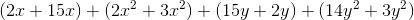Now, just combine like terms:Example Question #8 : Algebra

Simplify: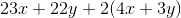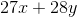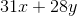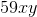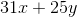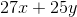Explanation:

You should begin by distributingthrough the whole group that it precedes: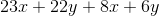Now, move your like variables next to each other: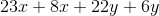Finally, combine the like terms:Example Question #9 : Algebra

Simplify: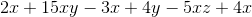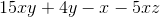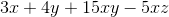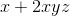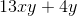Explanation:

First, group together your like variables: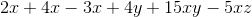The only like variables needing to be combined are the x-variables.  You can do this in steps or all at once: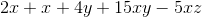Example Question #10 : Algebra

Simplify: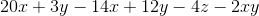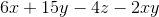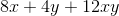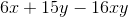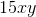Explanation:

First, move the like terms to be next to each other: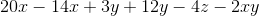Now, combine the x-variables and the y-variables:Example Question #1 : How To Add Variables

Simplify: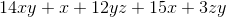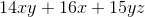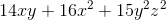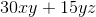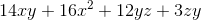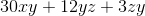Explanation:

Let's begin by moving the like terms toward each other.  Notice the following: zy is the same as yz.  (Recall the commutative property of multiplication.)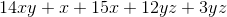Now, all you have to do is combine the x-variables and the yz-terms:Notice that you do not end up with any exponent changes.  That would only happen if you multiplied those variables.

Example Question #2 : How To Add Variables

Simplify: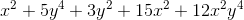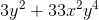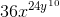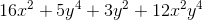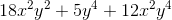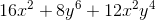Explanation:

Remember, when you have exponents like this, you will treat each exponented variable as though it were its own "type."  Likewise, pairs of variables are to be grouped together.  Therefore, group the problem as follows: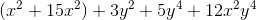Notice that the only thing to be combined are theterms.Example Question #3 : How To Add Variables

Simplify: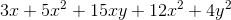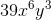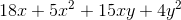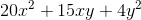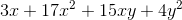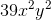Explanation:

Remember, for exponent problems, you group together different exponents and different combinations of variables as though each were a different type of variable.  Therefore, you can group your problem as follows: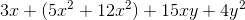Then, all you need to do is to combine theterms: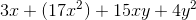← Previous 1 3 4 5 6